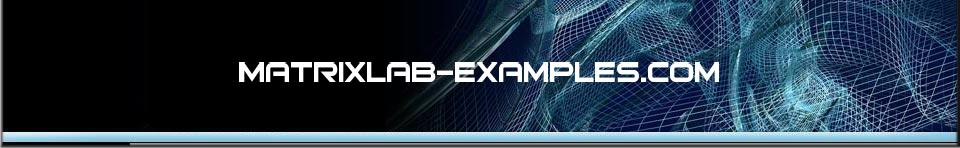# Boolean Algebra

These are the four logical operators for Boolean Algebra in Matlab.
It was named after George Boole, who first defined an algebraic system of logic in 19th. century.

 Boolean logic has many applications in electronics,  hardware and software, and is the basis of modern digital electronics. Boolean and Relational operators Summary In Matlab, there are four logical (aka boolean) operators. These operators produce vectors or matrices of the same size as the operands, with 1 when the condition is true, and 0 when the condition is false...

Laws of Boolean Algebra
In the following axioms and theorems, the '+' or 'V' signs represent a logical OR (or conjunction), the '.' or '^' signs represent a logical AND (or disjunction), and '¬' or '~' represent a logical NOT ( or negation)...

De Morgan's Laws
In Boolean Algebra, there are some very important laws which are called the De Morgan's laws. These laws teach us how to interchange NOT with AND or OR logical operators...

Logical AND. See details and examples
A & B performs a logical AND of arrays A and B and returns an array containing elements set to either logical 1 (TRUE) or logical 0 (FALSE).

Logical OR. See details and examples
A | B performs a logical OR of arrays A and B and returns an array   containing elements set to either logical 1 (TRUE) or logical 0 (FALSE).

Logical EXCLUSIVE OR. See details and examples
For the logical exclusive XOR(A,B), the result is logical 1 (TRUE) where either A or B, but not both, is nonzero.  The result is logical 0 (FALSE) where A and B are both zero or nonzero.

Logical NOT. See details and examples
~A performs a logical NOT of input array A, and returns an array containing elements set to either logical 1 (TRUE) or logical 0 (FALSE).

Combinational Logic
Combinational logic and sequential one. Its main difference is that the first type of circuit logic doesn’t depend on time or sequence of movements, and the second type of logic does. Let's code some gates...

From 'Boolean Algebra' to home